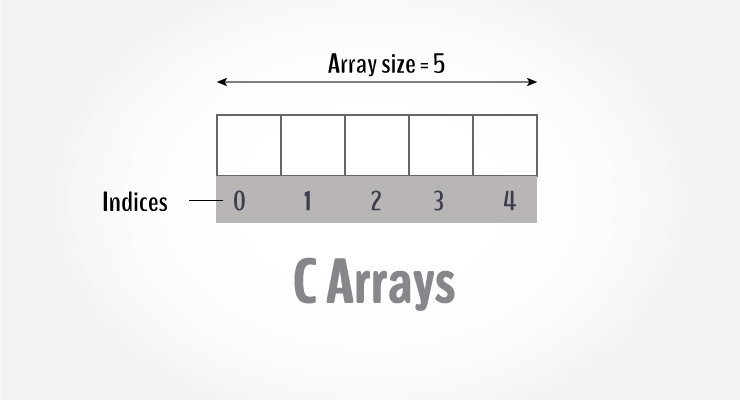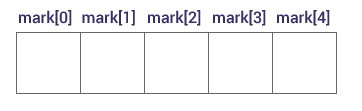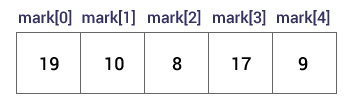# C Arrays

In this tutorial, you will learn to work with arrays. You will learn to declare, initialize and access elements of an array with the help of examples.Arrays in C

An array is a variable that can store multiple values. For example, if you want to store 100 integers, you can create an array for it.

````int data;`
```

## How to declare an array?

```dataType arrayName[arraySize];
```

For example,

`float mark;`

Here, we declared an array, mark, of floating-point type. And its size is 5. Meaning, it can hold 5 floating-point values.

It's important to note that the size and type of an array cannot be changed once it is declared.

## Access Array Elements

You can access elements of an array by indices.

Suppose you declared an array mark as above. The first element is mark, the second element is mark and so on.Declare an Array

#### Few keynotes:

• Arrays have 0 as the first index, not 1. In this example, mark is the first element.
• If the size of an array is n, to access the last element, the `n-1` index is used. In this example, mark
• Suppose the starting address of `mark` is 2120d. Then, the address of the `mark` will be 2124d. Similarly, the address of `mark` will be 2128d and so on.
This is because the size of a `float` is 4 bytes.

## How to initialize an array?

It is possible to initialize an array during declaration. For example,

``int mark = {19, 10, 8, 17, 9};``

You can also initialize an array like this.

``int mark[] = {19, 10, 8, 17, 9};``

Here, we haven't specified the size. However, the compiler knows its size is 5 as we are initializing it with 5 elements.Initialize an Array

Here,

```mark is equal to 19
mark is equal to 10
mark is equal to 8
mark is equal to 17
mark is equal to 9```

## Change Value of Array elements

``````int mark = {19, 10, 8, 17, 9}

// make the value of the third element to -1
mark = -1;

// make the value of the fifth element to 0
mark = 0;
``````

## Input and Output Array Elements

Here's how you can take input from the user and store it in an array element.

``````// take input and store it in the 3rd element
​scanf("%d", &mark);

// take input and store it in the ith element
scanf("%d", &mark[i-1]);```
```

Here's how you can print an individual element of an array.

``````// print the first element of the array
printf("%d", mark);

// print the third element of the array
printf("%d", mark);

// print ith element of the array
printf("%d", mark[i-1]);```
```

## Example 1: Array Input/Output

``````// Program to take 5 values from the user and store them in an array
// Print the elements stored in the array
#include <stdio.h>

int main() {
int values;

printf("Enter 5 integers: ");

// taking input and storing it in an array
for(int i = 0; i < 5; ++i) {
scanf("%d", &values[i]);
}

printf("Displaying integers: ");

// printing elements of an array
for(int i = 0; i < 5; ++i) {
printf("%d\n", values[i]);
}
return 0;
}```
```

Output

```Enter 5 integers: 1
-3
34
0
3
Displaying integers: 1
-3
34
0
3
```

Here, we have used a `for` loop to take 5 inputs from the user and store them in an array. Then, using another `for` loop, these elements are displayed on the screen.

## Example 2: Calculate Average

``````// Program to find the average of n numbers using arrays

#include <stdio.h>
int main()
{
int marks, i, n, sum = 0, average;

printf("Enter number of elements: ");
scanf("%d", &n);

for(i=0; i<n; ++i)
{
printf("Enter number%d: ",i+1);
scanf("%d", &marks[i]);

// adding integers entered by the user to the sum variable
sum += marks[i];
}

average = sum/n;
printf("Average = %d", average);

return 0;
}```
```

Output

```Enter n: 5
Enter number1: 45
Enter number2: 35
Enter number3: 38
Enter number4: 31
Enter number5: 49
Average = 39
```

Here, we have computed the average of n numbers entered by the user.

### Access elements out of its bound!

Suppose you declared an array of 10 elements. Let's say,

`int testArray;`

You can access the array elements from `testArray` to `testArray`.

Now let's say if you try to access `testArray`. The element is not available. This may cause unexpected output (undefined behavior). Sometimes you might get an error and some other time your program may run correctly.

Hence, you should never access elements of an array outside of its bound.

## Multidimensional arrays

In this tutorial, you learned about arrays. These arrays are called one-dimensional arrays.

In the next tutorial, you will learn about multidimensional arrays (array of an array).